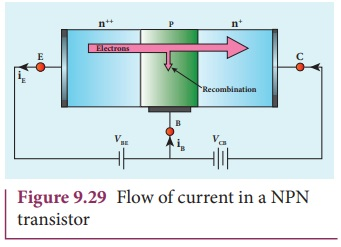Home | | Physics 12th Std | Transistor action in the common base mode

# Transistor action in the common base mode

The operation of an NPN transistor in the common base mode is explained below.

Transistor action in the common base mode

The operation of an NPN transistor in the common base mode is explained below. The current flow in a common base NPN transistor in the forward active mode is shown in Figure 9.29.Basically, a BJT can be considered as two p-n junction diodes connected back- to-back. In the forward active bias of the transistor, the emitter-base junction is forward biased by a dc power supply VEB and the collector-base junction is reverse  biased by the bias power supply VCB. The forward bias decreases the depletion region across the emitter-base junction and the reverse bias increases the depletion region across the collector-base junction. Hence, the barrier potential across the emitter-base junction is decreased and the collector-base junction is increased. The voltage across the emitter-base junction is represented as VEB and the collector-base junction as VCB.

In an NPN transistor, the majority charge carriers in the emitter are electrons. As it is heavily doped, it has a large number of electrons. The forward bias across the emitter- base junction causes the electrons in the emitter region to flow towards the base region and constitutes the emitter current (IE) . The electrons after reaching the base region recombine with the holes in the base region. Since the base region is very narrow and lightly doped, all the electrons will not have sufficient holes to recombine and hence most of the electrons reach the collector region.

Eventually, the electrons that reach the collector region will be attracted by the collector terminal as it has positive potential and flows through the external circuit. This constitutes the collector current (IC ) . The holes that are lost due to recombination in the base region are replaced by the positive potential of the bias voltage VEE and constitute the base current (IB) . The magnitude of the base current will be in micoamperes as against milliamperes for emitter and collector currents.

It is to be noted that if the emitter current is zero, then the collector current is almost zero. It is therefore imperative that a BJT is called a current controlled device. Applying Kirchoff ŌĆÖs law, we can write the emitter current as the sum of the collector current and the base current.

IE = IB + ICSince the base current is very small, we can write, IE Ōēł IC . There is another component of collector current due to the thermally generated electrons called reverse saturation current, denoted as (ICO ). This factor is temperature sensitive. Therefore, care must be taken towards the stability of the system at high temperatures.

The ratio of the collector current to the emitter current is called the forward current gain (╬▒dc ) of a transistor.

╬▒dc = IC / IE

The ╬▒ of a transistor is a measure of the quality of a transistor. Higher the value of ╬▒ better is the transistor. It means that the collector current is closer to the emitter current. The value of ╬▒ is less than unity and ranges from 0.95 to 0.99. This indicates that the collector current is 95% to 99% of the emitter current.

1. The conventional flow of current is based on the direction of the motion of holes

2. In NPN transistor, current enters from the base into the emitter.

3. In a PNP transistor, current enters from the emitter into the base.

4. The emitter-base junction has low resistance and the collector-base junction has high resistance.

## Working of a PNP transistor

The working of a PNP transistor is similar to the NPN transistor except for the fact that the emitter current IE is due to holes and the base current IB is due to electrons. However, the current through the external circuit is due to the flow of electrons.

### EXAMPLE 9. 5

In a transistor connected in the common base configuration, ╬▒=0.95 , IE =1 mA . Calculate the values of IC and IB .

### Solution

╬▒= IC/IE

IC =╬▒ IE =0.95├Ś1=0.95 mA

IE = IB + IC

Ōł┤ IB = IC ŌłÆIE =1ŌłÆ0.95=0.05 mA

Tags : Bipolar Junction Transistor [BJT] , 12th Physics : UNIT 10a : Semiconductor Electronics
Study Material, Lecturing Notes, Assignment, Reference, Wiki description explanation, brief detail
12th Physics : UNIT 10a : Semiconductor Electronics : Transistor action in the common base mode | Bipolar Junction Transistor [BJT]